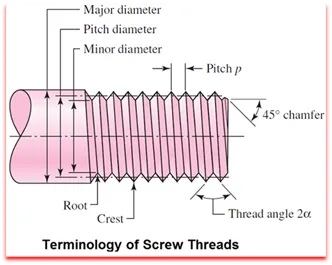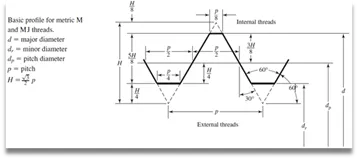Screw thread is a continuous helical groove of specified cross-section produced on the external or internal surface. A screw thread formed on a cylinder is known as straight or parallel screw thread, while screw thread formed on a cone or frustum is known as tapered screw thread.Axis of a thread: This is a imaginary line running longitudinally through the centre of the screw.
Crest: Crest of thread is the top most surface joining the two sides.
Root: Root of thread is the bottom of the groove between the two flanks.
Flank: Flank of thread are straight edges which connect the crest with root of thread.
Pitch: Pitch of a thread is the distance measured parallel to the axis from a point on a thread to the corresponding points on adjacent thread forms in the same axial plane and on the same side of axis.
Depth of thread: Depth of a thread is the distance between the crest and root of the thread.
Major diameter: It is an imaginary largest diameter of thread which would touch the crests of internal or external thread.
Minor diameter: It is an imaginary smallest diameter of thread which would touch the roots of an external thread.
Pitch diameter: It is a theoretical diameter between the major and minor diameter of screw threads.Helix angle: On straight thread, It is the angle made by the helix of the thread at the pitch line with the axis.
Lead angle: On straight thread, It is the angle made by the helix of the thread at the pitch line with plane perpendicular to the axis. Lead angle is measured in an axial plane.
Flank angle: Flank angle is the angle made by the flank of a thread with the perpendicular to the axis of a thread.
Included angle: Included angle is the angle between the flanks or slope of the thread measured in an axial plane.
The lead: It is the distance the nut moves parallel to the screw axis when the nut is given one turn. For a single thread as shown in the figure above, the lead is the same as the pitch of the screw thread.